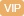| APP | 校园号 | 客服 客服热线：400-863-9889

400-863-9889A．1 B．2 C．3
【考点】整数的乘法及应用【分析】根据式的性质，边同加上
 4 5
x，得
 3 4
+
 4 5
x=
 42 25
，两边同去
 3 4
，再乘
 5 4

 3 21
x=
 6 7
×
 9 35
，即
 1 7
x=
 6 7
×
 9 35
，边同乘7可；

 1 2
，再同除以可；

 9 4
x=
 27 16
，根据等式的，两边同乘
 4 9

 1 10
x=0.36，根据等的质两同乘0即可．
【解答】解：
 7 25
×6-
 4 5
x=
 3 4

（1+x）×4=2×5
2x-
 1 2
+
 1 2
=0.+
 1 2

1+x=25

 4 5
x=
 93 100

=15
2x=1
1x-1=2.5-1
2÷2=1÷2
x+
 5 4
x=
 27 16

（1+）×4÷=2×54
=
 93 80

 1 10
x10=0.36×0
2x-
 1 2
=05

 9 4
 4 9
=
 27 16
×
 4 9

 4 5
 5 4
=
 93 100
×
 5 4

 1 10
x=0.6
x=
 54 35

 3 4
+
 4 5
x=
 42 25

x=
 3 4

x=
 1 2

2（1+x）=
 4 5

x=3.6
【点评】题要考了根据等式的性质解方的能力等两同时加上或时减去、时乘以或同除以一个数（0除外），两仍相等．

0/0